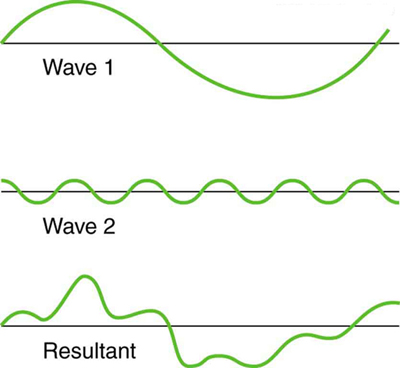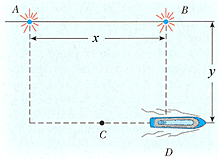## Physics Tutorial

#### Intro

Waves have the unique property that they can simultaneously exist in the same place. When two waves occupy the same space, their functions are added together.

When waves have opposite signed values when they pass through each other, they cancel out. This is known as destructive interference.When waves have same signed values when they pass through each other, they add together in magnitude. This is known as constructive interference.When waves are not out of phase by a multiple of one half of their wave length, or have different wavelengths, new waveforms are created. This is the principle behind Fourier Analysis.#### Sample Problem

The ship in the figure travels along a straight line parallel to the shore and y = 580 m from the shore. The ship’s radio receives simultaneous signals of the same frequency from antennas at points A and B, which are x = 740 m apart. The signals interfere constructively at point C, which is equidistant from A and B. The signal goes through the first minimum at point D. Determine the wavelength of the radio waves.Source: College Physics by Serway and Faughn

#### Solution

Let’s draw a right triangle between points A, B, and D.

`A____B`
`\    |`
` \   |`
`  \  |`
`   \ |`
`    \|`
`      D`

What else do we know?
We know that the distance CD = x = 740m, and AD = y = 580m.
We also know that the wavelengths of the radio waves from the each of the two towers are the same.

At point D, The signal from tower A will have traveled the hypotenuse length AD, while the signal from tower B will have traveled only BD.
Since the signal is at a minimum at D and the signals from each tower have the same wavelength, the difference between the distances that the two signals travel must be a multiple of one half of their wavelength (see the destructive interference picture).
Moreover, since point D is the first minimum, it must be the first time that the signals destructively interfere, which means that the difference in the distances the two signals travel must be exactly 1 times one half of their wavelength.

The distance the signal from tower A travels, AD = sqrt(AB^2 + BD^2) = sqrt(740^2 + 580^2) = 940 meters.
The distance the signal from tower B travels is BD = 580 meters.

Subtracting AD from AC to get one half the wavelength: 940 – 580 = 360 meters.

Multiplying by two to find the full wavelength: 360*2 = 720 meters.

That’s all folks and I’ll gladly see you next time.